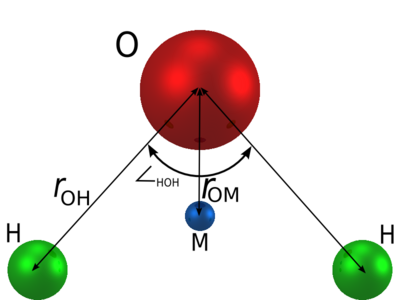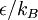2022: SklogWiki celebrates 15 years on-line

TIP4P/ε water model

(Redirected from TIP4P/epsilon)$r_{\mathrm {OH}}$ (Å)$\angle$HOH , deg$\sigma$ (Å)$\epsilon/k_B$ (K) q(O) (e) q(H) (e) q(M) (e)$r_{\mathrm {OM}}$ (Å) 0.9572 104.52 3.165 93.0 0 0.527 -2q(H) 0.105